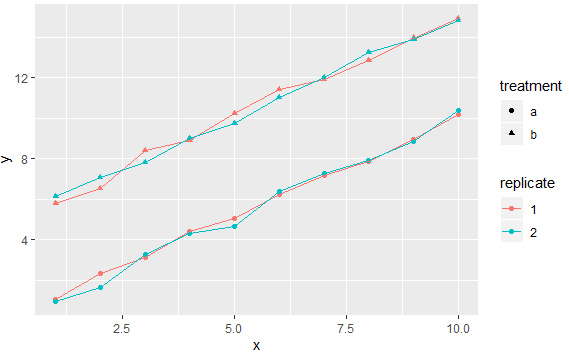2 views

Is it possible to group by two columns? So the cross product is drawn by geom_point() and geom_smooth()?

As example:

frame <- data.frame(series <- rep(c('a', 'b'), 6), sample <- rep(c('glass',

'water', 'metal'), 4), data <- c(1:12))

ggplot(frame, aes()) # ...

Such that the points 6 and 12 share a group, but not with 3.

by

To group two columns as a new factor in ggplot2, you can use the interaction function from the base package as follows:

set.seed(123)

x <- rep(1:10,4)

y <- c(rep(1:10, 2)+rnorm(20)/5, rep(6:15, 2) + rnorm(20)/5)

treatment <- gl(2, 20, 40, labels=letters[1:2])

replicate <- gl(2, 10, 40)

d <- data.frame(x=x, y=y, treatment=treatment, replicate=replicate)

ggplot(d, aes(x=x, y=y, colour=replicate, shape = treatment,

group=interaction(treatment, replicate))) +

geom_point() + geom_line()

Output: